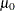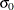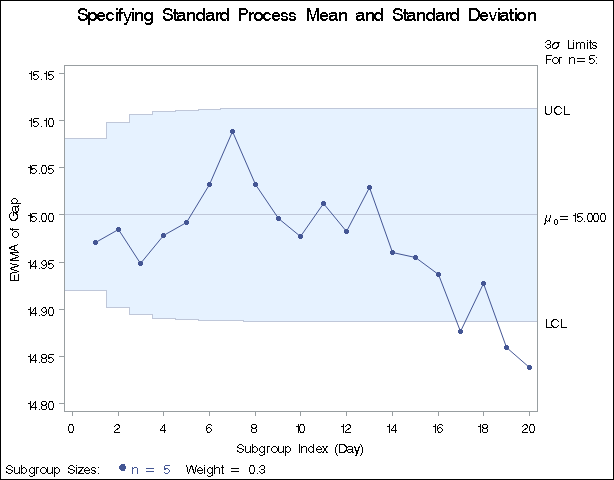#### Example 9.1 Specifying Standard Values for the Process Mean and Process Standard Deviation

See MACEW2 in the SAS/QC Sample LibraryBy default, the EWMACHART statement estimates the process mean () and standard deviation () from the data. This is illustrated in the Getting Started section of this chapter. However, there are applications in which standard values (and) are available based, for instance, on previous experience or extensive sampling. You can specify these values with the MU0= and SIGMA0= options.

For example, suppose it is known that the metal clip manufacturing process (introduced in Creating EWMA Charts from Raw Data) has a mean of 15 and standard deviation of 0.2. The following statements specify these standard values:

ods graphics off;
title 'Specifying Standard Process Mean and Standard Deviation';
symbol v=dot h=0.8;
proc macontrol data=Clips1;
ewmachart Gap*Day /
mu0     = 15
sigma0  = 0.2
weight  = 0.3
xsymbol = mu0;
run;


The XSYMBOL= option specifies the label for the central line. The resulting chart is shown in Output 9.1.1.

Output 9.1.1: Specifying Standard Values with MU0= and SIGMA0=The central line and control limits are determined usingand(see the equations in Table 9.3). Output 9.1.1 indicates that the process is out-of-control, since the moving averages for Day=17, Day=19, and Day=20 lie below the lower control limit.

You can also specifyandwith the variables _MEAN_ and _STDDEV_ in a LIMITS= data set, as illustrated by the following statements:

data Cliplim;
length _var_ _subgrp_ _type_ \$8;
_var_    = 'Gap';
_subgrp_ = 'Day';
_type_   = 'STANDARD';
_limitn_ = 5;
_mean_   = 15;
_stddev_ = 0.2;
_weight_ = 0.3;

proc macontrol data=Clips1 limits=Cliplim;
ewmachart Gap*Day / xsymbol=mu0;
run;


The variable _WEIGHT_ is required, and its value provides the weight parameter used to compute the EWMAs. The variables _VAR_ and _SUBGRP_ are also required, and their values must match the process and subgroup-variable, respectively, specified in the EWMACHART statement. The bookkeeping variable _TYPE_ is not required, but it is recommended to indicate that the variables _MEAN_ and _STDDEV_ provide standard values rather than estimated values.

The resulting chart (not shown here) is identical to the one shown in Output 9.1.1.